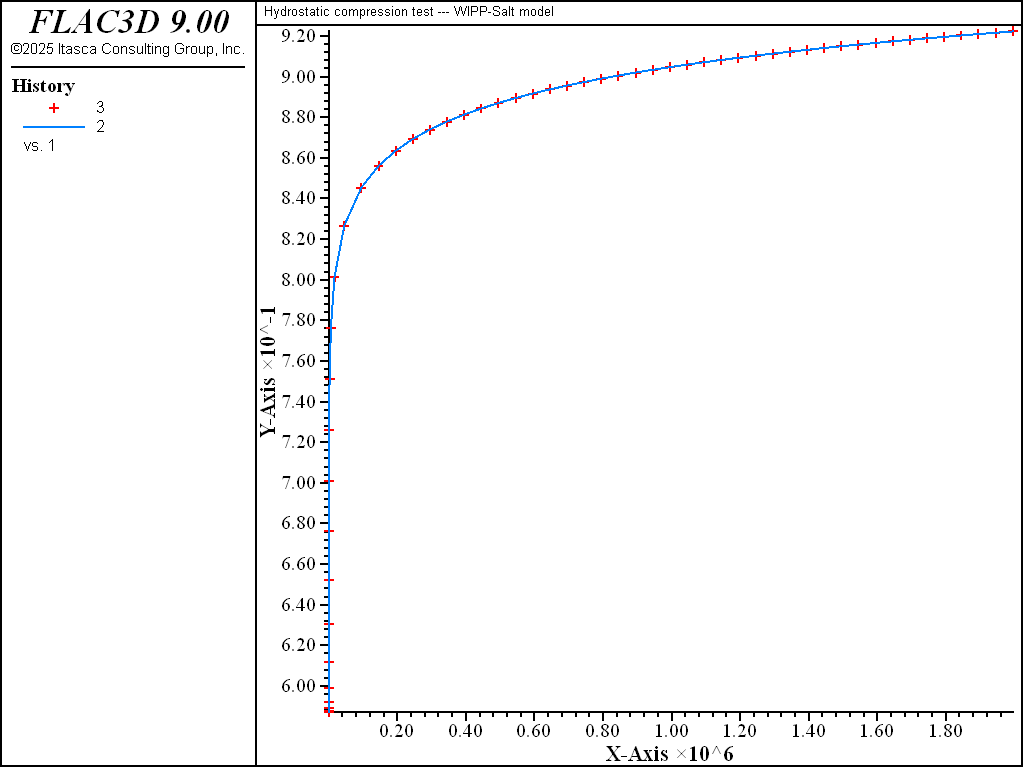# WIPP-Salt Model: Hydrostatic Compression Test

Note

To view this project in FLAC3D, use the menu command Help ► Examples…. Choose “Creep/HydrostaticCompressionWIPPSalt” and select “HydrostaticCompressionWIPPSalt.prj” to load. The project’s main data files are shown at the end of this example.

Results of the hydrostatic compression test are presented here to validate the WIPP-Salt model in FLAC3D. The test involves one zone at an initially elastic state of equilibrium. The zone is allowed to creep for a period of 23 days, during which time the fractional density is monitored and compared to the analytical calculation.

In this hydrostatic compression test, the confining pressure is held constant. The rate of change of density (see WIPP-Salt model) is

(1)$\dot{\rho} = B_0 \left[e^{-B_1 \sigma} - 1 \right] e^{B_2 \rho}$

Note that $$B_0 \left[e^{-B_1 \sigma} - 1 \right]$$ is constant for this problem.

Using definition $$F_d = \rho / \rho_f$$ (see WIPP-Salt model) for the fractional density, and given that $$\rho = \rho_{\circ}$$ at $$t$$ = 0, integration of Equation (1) yields

(2)$F_d = - {{1}\over{B_2 \rho_f}} ln \biggl[-B_2 B_0 \left[e^{-B_1 \sigma} - 1 \right]t + e^{-B_2 \rho_{\circ}} \biggr]$

where $$\rho_f$$ is the density of intact salt.

Also, integrating $$\dot{\epsilon}_v = -\dot{\rho}/\rho$$ (see WIPP-Salt model), we obtain, for zero initial strain,

(3)$\epsilon_v = - ln {{\rho}\over{\rho_{\circ}}}$

Numerical results for fractional density versus creep time are compared to the analytical calculations in Figure 1 for a stress level of 8.97 MPa. The initial density is 1350 kg/m3, and the final density is 2300 kg/m3.Figure 1: Comparison of numerical and analytical prediction for fractional density.

Data File

;------------------------------------------------------
;       Hydrostatic Compression test -- WIPP-Salt model
;------------------------------------------------------
model new
fish automatic-create off
model title 'Hydrostatic compression test --- WIPP-Salt model'
model configure creep
; --- geometry ---
zone create brick size 1 1 1 edge 5
zone face skin
program call 'parameter'
[cons]
; --- constitutive model ---
zone cmodel assign wipp-salt
zone property bulk 0.1186e9 shear 0.0714e9 activation-energy 12000 ...
constant-a 4.56 constant-b 127 constant-d 5.79e-36 ...
creep-rate-critical 5.39e-8 exponent 4.9 temperature 300 ...
constant-gas 1.987 bulk-final [valbf] shear-final [valsf] ...
density-final [valrhof] compaction-0 [valb0] ...
compaction-1 [valb1] compaction-2 [valb2] density-salt [valrho]
; --- boundary conditions ---
zone face apply velocity-norm 0.0   range group 'Bottom'
zone face apply velocity-norm 0.0   range group 'West'
zone face apply velocity-norm 0.0   range group 'South'
zone face apply stress-norm [valsig] range group 'Top'
zone face apply stress-norm [valsig] range group 'East'
zone face apply stress-norm [valsig] range group 'North'
; --- initial conditions ---
zone initialize density [valrho]
zone initialize stress xx [valsig] yy [valsig] zz [valsig]
; --- histories ---
model history creep time-total
fish history c_frd
fish history c_frdsol
fish history c_err
fish history c_ev
fish history c_evsol
fish history c_rbulk
; --- test ---
model large-strain on
model creep active on
model creep timestep starting 5.e-5
model creep timestep minimum 5.e-5 maximum 500.
model solve time-total 2e6
model save 'hyd.sav'